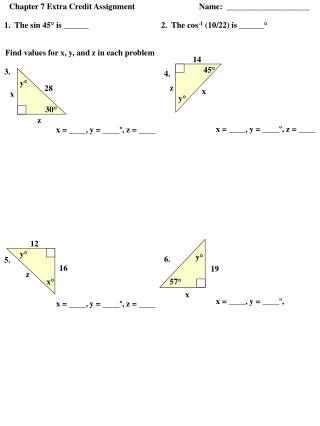DownloadDownload PresentationFind values for x, y, and z in each problem

# Find values for x, y, and z in each problem

Télécharger la présentation## Find values for x, y, and z in each problem

- - - - - - - - - - - - - - - - - - - - - - - - - - - E N D - - - - - - - - - - - - - - - - - - - - - - - - - - -
##### Presentation Transcript

1. Chapter 7 Extra Credit Assignment Name: ____________________ 14 45° 3. 4. y° z 28 x x y° 30° z 12 y° y° 6. 5. 16 19 z 57° x° x 1. The sin 45° is ______ 2. The cos-1 (10/22) is ______° Find values for x, y, and z in each problem x = ____, y = ____º, z = ____ x = ____, y = ____º, z = ____ x = ____, y = ____º, x = ____, y = ____º, z = ____

2. z 8. x° 7. z 31 9 15 x° 43 x 9. 60° 10. y° 26 x z 38 y° 33° y° y° x = ____, y = ____º, z = ____ x = ____, y = ____º, z = ____ z x = ____, y = ____º, z = ____ x = ____, y = ____º, z = ____

3. Solve each problem. Round measures of segments to the nearest tenth and measures of angles to the nearest degree. 11. From the top of a tower, the angle of depression to a stake on the ground is 72 degrees. The top of the tower is 80 feet above ground. How far is the stake from the foot of the tower? 12. A tree 40 feet high casts a shadow 58 feet long. Find the measure of the angle of elevation of the sun. 13. A ladder leaning against a house makes an angle of 60 degrees with the ground. The foot of the ladder is 7 feet from the foot of the house. How long is the ladder? 14. A balloon on a 40 foot string makes an angle of 50 degrees with the ground. How high above the ground is the balloon if the hand of the person holding the balloon is 6 feet above the ground? 15. A 20 foot ladder leans against a wall so that the base of the ladder is 8 feetfrom the base of the building. What angle does the ladder make with the ground?

4. 16. A 50 meter vertical tower is braced with a cable secured at the top of the tower and tied 30 meters from the base. What angle does the cable form with the vertical tower? 17. At a point on the ground 50 feet from the foot of a tree, the angle of elevation to the top of the tree is 53 degrees. Find the height of the tree. 18. From the top of a lighthouse 210 feet high, the angle of depression of a boat is 27 degrees. Find the distance from the boat to the foot of the lighthouse if the lighthouse is at sea level. 19, Richard is flying a kite. The kite string makes an angle of 57 degrees with the ground. If Richard is standing 100 feet from the point on the ground directly below the kite, find the length of the kite string. 20. An airplane rises vertically 1000 feet over a horizontal distance of 1 mile. What is the angle of elevation of the airplane's path?